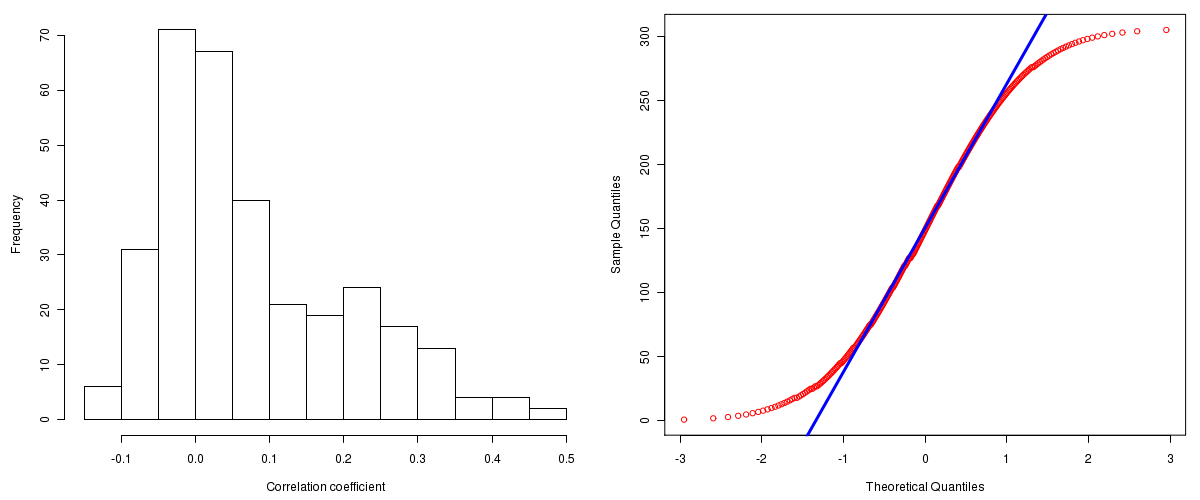Glioblastoma Multiforme: Correlations between copy number and miR expression
Maintained by John Zhang (Dana-Farber Cancer Institute)
Overview
Introduction

A TCGA sample is profiled to detect the copy number variations and expressions of genes. This pipeline attempts to correlate copy number and expression data of genes across samples to determine if the copy number variations also result in differential expressions. This report contains the calculated correlation coefficients based on measurements of genomic copy number (log2) values and intensity of the expressions of the corresponding feature across patients. High positive/low negative correlation coefficients indicate that genomic alterations result in differences in the expressions of miRNA the genomic regions transcribe.

Summary

The correlation coefficients in 10, 20, 30, 40, 50, 60, 70, 80, 90 percentiles are -0.05784, -0.02672, -0.00676, 0.01442, 0.0362, 0.0664, 0.11348, 0.19932, 0.27524, respectively.

Results
Correlation results

Number of genes and samples used for the calculation are shown in Table 1. Figure 1 shows the distribution of calculated correlation coefficients and quantile-quantile plot of the calculated correlation coefficients against a normal distribution. Table 2 shows the top 20 features ordered by the value of correlation coefficients.

Table 1.  Counts of miR and number of samples in copy number and expression data sets and common to both

Category Copy number Expression Common
Sample 537 491 433
miR 357 535 357

Figure 1.  Summary figures. Left: histogram showing the distribution of the calculated correlations across samples for all miR. Right: QQ plot of the calculated correlations across samples. The QQ plot is used to plot the quantiles of the calculated correlation coefficients against that derived from a normal distribution. Points deviating from the blue line indicate deviation from normality.Table 2.  Get Full Table Top 20 features (defined by the feature column) ranked by correlation coefficients

feature r p-value q-value chrom start end geneid
hsa-miR-339 0.4776 0 0 7 1029095 1029188 MI0000815
hsa-miR-491 0.4624 0 0 9 20706104 20706187 MI0003126
hsa-miR-125a 0.4311 0 0 19 56888319 56888404 MI0000469
hsa-miR-99b 0.4171 0 0 19 56887677 56887746 MI0000746
hsa-let-7b 0.4155 0 0 22 44888230 44888312 MI0000063
hsa-miR-148a 0.4016 0 0 7 25956064 25956131 MI0000253
hsa-miR-148b 0.3953 0 0 12 53017267 53017365 MI0000811
hsa-miR-151 0.3703 1.55431223447522e-15 4.04928946797407e-14 8 141811845 141811934 MI0000809
hsa-miR-23b 0.3634 5.77315972805081e-15 1.33690826879144e-13 9 96887311 96887407 MI0000439
hsa-miR-130b 0.355 2.62012633811537e-14 5.46075608252503e-13 22 20337593 20337674 MI0000748
hsa-miR-135b 0.3494 7.061018436616e-14 1.33784316967871e-12 1 203684053 203684149 MI0000810
hsa-miR-590 0.3479 9.2148511043888e-14 1.60043345638975e-12 7 73243464 73243560 MI0003602
hsa-miR-186 0.3437 1.89182003396127e-13 3.03296230919904e-12 1 71305902 71305987 MI0000483
hsa-miR-377 0.3412 2.88657986402541e-13 4.29720514968677e-12 14 100598140 100598208 MI0000785
hsa-miR-15a 0.3369 5.91748872125208e-13 8.22198585306735e-12 13 49521256 49521338 MI0000069
hsa-miR-100 0.3345 8.91287044169076e-13 1.16098913746057e-11 11 121528147 121528226 MI0000102
hsa-miR-320 0.331 1.56141766183282e-12 1.91425906143151e-11 8 22158420 22158501 MI0000542
hsa-miR-127 0.3193 1.01274544306307e-11 1.17262281037649e-10 14 100419069 100419165 MI0000472
hsa-let-7e 0.3169 1.48234757801902e-11 1.62602415393974e-10 19 56887851 56887929 MI0000066
hsa-miR-27b 0.3161 1.67978964071835e-11 1.75047312880975e-10 9 96887548 96887644 MI0000440
Methods & Data
Input

Gene level (TCGA Level III) expression data and copy number data of the corresponding loci derived by using the CNTools package of Bioconductor were used for the calculations. Pearson correlation coefficients were calculated for each pair of genes shared by the two data sets across all the samples that were common.

Correlation across sample

Pairwise correlations between the log2 copy numbers and expressions of each gene across samples were calculated using Pearson correlation.